Rating : ⭐⭐⭐⭐⭐
Price : \$10.99
Language:EN
Pages: 232

# Disk average the mean service times are for the cpu

Performance Analysis

Peter Harrison & Giuliano Casale

▶ Lectures: Wednesdays 9:00–11:00, in 144; Tutorials: Wednesdays 11:00–12:00, in 144.

▶ 18 lectures, 9 tutorials, 2 pieces of assessed coursework.

Example 1: A simple transaction processing (TP) server

A transaction processing (TP) system accepts and processes a

service rate

Q: If both the arrival rate and service rate are doubled, what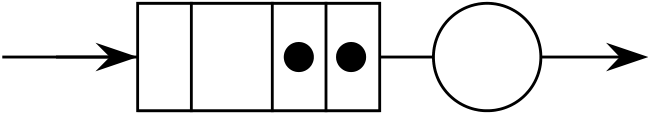▶ The arrival rate is 15tps

Example 3: A simple multiprocessor TP system
Consider our TP system but this time with multiple transaction processors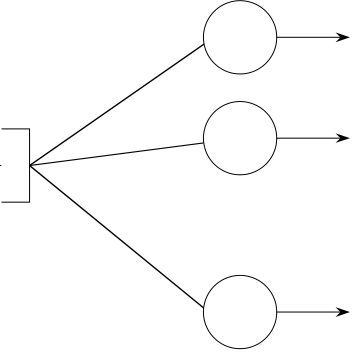∝ Possion arrival
process, rate λ  ∝

∝
m parallel TP servers

▶ The arrival rate is 16.5 tps
▶ The mean service time per transaction is 58.37ms
Q: By how much is the system response time reduced by adding one processor?

q
∝1

γ

6 / 226

Example 5: A simple computer model
Consider an open uniprocessor CPU system with just disks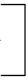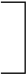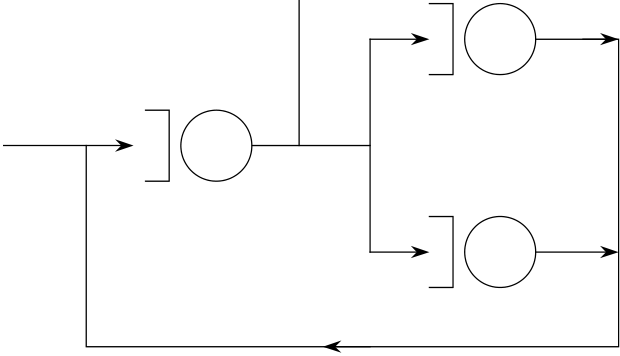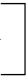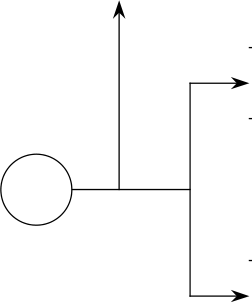1
2
q10

Disk1

∝1

3
λ

▶ Each submitted job makes 121 visits to the CPU, 70 to disk 1 and 50 to disk 2 on average
▶ The mean service times are 5ms for the CPU, 30ms for disk 1 and 37ms for disk 2

Q: What is the effect of replacing the CPU with one twice the speed?

1 q11

q12

∝2 q21
Disk1

∝1

CPU q13 3 ∝3 q31

8 / 226

Example 7: A multiprogramming system with virtual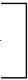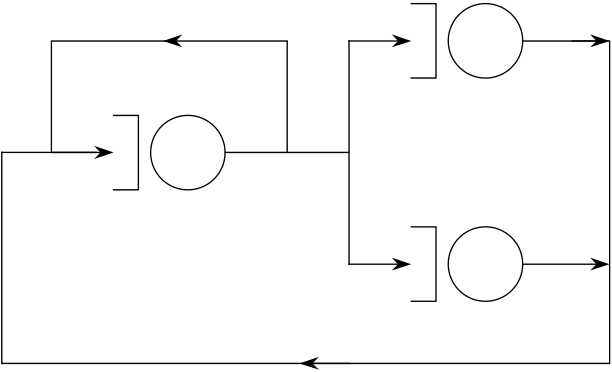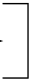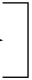▶ Each job page faults at a rate determined by the following lifetime function:

Life-time function example

Mean time between faults 0.7

1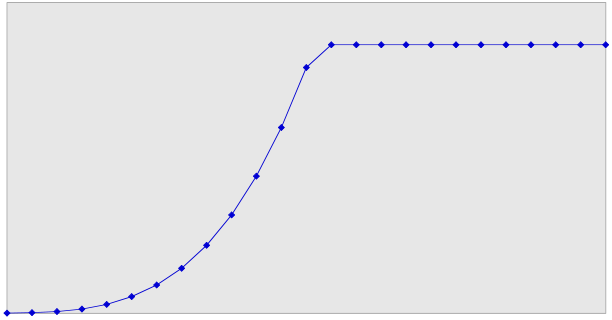6

0.6
0.5
0.4
0.3
0.2
0.1
3.0 6.0 9.0 12.0 15.0 18.0

Q: What number of batch jobs keeps the system throughput at its maximum and at what point does thrashing occur?

10 / 226

K

:

Disk 1

Introduction

Computer systems are

We also see these characteristics in queues of customers in a bank

or supermarket, or prices on the stock exchange.

{Xt ∈|t ∈ T}, each defined on some sample space Ω (the same for each) for a parameter space T.

T and Ω may be either discrete or continuous

T is normally regarded as time

The Poisson process is a renewal process with renewal period

(interarrival time) having cumulative distribution function F and

14 / 226

Memoryless property of the (negative) exponential distribution

15 / 226

Proof.

=e−λt − e−λ(t+s) e−λt

= 1 − e−λs

▶ e.g. when you get to a bus stop, how long will you have to wait for the next bus?

▶ If the renewal process is Poisson, R has the same distribution as S by the memoryless property

∀t

Therefore

▶ From it we can derive the distribution function of the
interarrival times (i.e. negative exponential) and the Poisson distribution for Nt (the number of arrivals in time t)

P(Nt = n) = (λt)n n!

Derivation of the interarrival time distribution

P(S > t + h) = P = P(S > t)P(no arrival in (t, t + h])�(S > t) (no arrival in (t, t + h])�

by the memoryless property. Let G(t) = P(S > t). Then: G(t + h) = G(t)P(no arrival in (t, t + h]) = (1 − hλ)G(t) + o(h)

and so

G(t) = ke−λt

How It Works